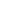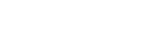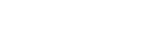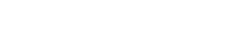# Binomial Coefficient

Binomial coefficients tell us how many ways there are to choose k things out of larger set. More formally, they are defined as the coefficients for each term in (1+x)n. Written as, (read n choose k), whereis the binomial coefficient of the xk term of the polynomial.

An alternate notation is nCk.

For non-negative integer values of n (number in the set) and k (number of items you choose), every binomial coefficient nCk is given by the formula:The “!” symbol is a factorial.

## Examples

Imagine you have 5 elements {a, b, c, d, f}. To find out how many different subsets of 2 elements it has, look at the binomial coefficient 5C2.This equals 10.

For a more detailed explanation of how to solve a formula like this, watch the following video:

Watch this video on YouTube.

For a more concrete example, suppose the president of a student club must pick three members of an advisory board from a faculty pool of 24. To find out how many ways he could make this choice, look at the 24C3 binomial coefficient, 24!/(3! 21!)= 2024. So the president of the student club has 2024 cabinet choices.

## Importance of the Binomial Coefficient in Statistics

The binomial coefficient is much more than just a simple formula to calculate how many ways you can pull an advisory board from a candidate pool, a 4-digit pin from a 10-digit set, or a plate of apples from a bin of the same. It’s also part of the description of the binomial distribution, a simple probability distribution for frequently encountered 2-outcome situations.

If your observations are independent, each represents one of two outcomes (think: success and failure), your number of trials are fixed and the probability of success is the same for each trial, then the probability you have exactly r successes during your n independent trials will beThis formula represents the binomial distribution. Here p is the probability of success in each instance, and q=1-p, the probability of failure.

The binomial coefficient n choose r tells you how many success-failure sequences, of the set of all possible sequences, will result in exactly r successes.  The probability of each of those individual sequences happening is just  prqn-r.

## References

Binomial Coefficients @ Dartmouth. Retrieved September 27, 2017 from: https://math.dartmouth.edu/archive/m19w03/public_html/Section1-3.pdf
CS4205 Binomial Coefficients. Retrieved September 27, 2017 from: http://www.cs.columbia.edu/~cs4205/files/CM4.pdf

CITE THIS AS:
Stephanie Glen. "Binomial Coefficient" From StatisticsHowTo.com: Elementary Statistics for the rest of us! https://www.statisticshowto.com/binomial-coefficient/
---------------------------------------------------------------------------Need help with a homework or test question? With Chegg Study, you can get step-by-step solutions to your questions from an expert in the field. Your first 30 minutes with a Chegg tutor is free!